The Nature of Light: Source's Frame of Reference

Suppose light were part of the essence of its source dispersing itself thro­ugh space. Then it would be sensible to consider the velocity of light as be­ing relative only to the frame of reference of its source. [Português]

Classically, it was assumed that light must travel as a progressive disturbance with­in the universal frame of reference of a luminiferous aether. This idea was discred­ited because light appeared to traverse the space between any source and any ob­server at a universally fixed velocity c. This velocity appeared to be independent of the relative velocities of the source, observer and the rest of the universe. It was subsequently theorized that light always travelled at its universally fixed velocity c from the point of view of any observer who was moving with a constant velocity. We have already explored this observer centred view of the propagation of light in the previous essay.

However, the idea that the velocity of light appears to be the same from the points of view of all observers who are moving with constant velocities is also problematic. This is because the apparent distance light travels, in going from its source to an observer, differs according to whether we consider the process from the point of view of the source or from the point of view of the observer. The same is true for the time it takes. This problem was circumvented by postulating that space be­comes contracted, to different extents, within the frames of reference of different observers according to their relative velocities with respect to the light-source. This postulation also required that time became correspondingly dilated.

Notwithstanding, I believe that there is a much more plausible way of interpreting observation that should be considered. Let us first look again at the passage of light between source and observer from the point of view of an arbitrary observer.

The Observer-Centred View

An observer is observing a source of light. It is moving away from him at a sign­ificant fraction v of the velocity of light. In free space we know that velocity is rela­tive. Consequently, it should not matter whether we think of the observer as "sta­tionary" and the light-source as "moving" or whether we think of the light-source as "stationary" and the observer as "moving". Whichever of these two points of view we choose should, in principle, make no difference to the way light travels between the light-source and the observer. If we accept that light travels with velocity c in all frames of reference in uniform motion, then for any observer of the light-source, these two points of view will be as follows.

1) Moving Light-Source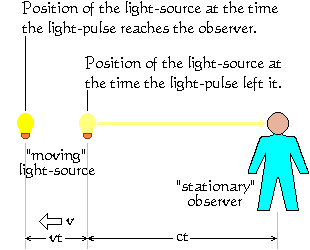The case where we consider the source of light to be moving is shown on the right. A pulse of light travels from the position in the observer's frame of reference where it was emitted by the light-source. It tra­vers­es the space from that point to where the observer is located at the "origin" of the coordinate system of his frame of ref­er­en­ce. It traverses this space at velocity c in time t. The distance it travels is there­fore ct. During the time t, the "moving" light-source travels a distance vt further from the observer, where v is the relative velo­city between the obser­ver and the light-source. At the time the light pulse reaches the observer, the observer will see it as having come from the posi­tion where the light-source was when it emitted the light-pulse.

The consequences of this are as follows. The observer sees only a phantom of the light-source as it was and where it was at an amount of time t into the past. The observer has no way of being able to see the light-source as it is "now" and where it is "now". By the term "now" I mean "at the instant the observer is receiving the light". The distance at any given instant between the observer and the actual real­ity of the light-source is (v + c)t.

2) Moving Observer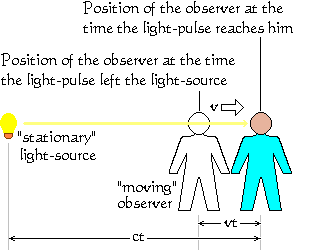On the left is shown the reverse situation where we consider the light-source to be "stationary" and the observer to be "mov­ing". A light-pulse is emitted by the light-source. It travels at velocity c towards the observer. While the light-pulse is travelling towards the observer, the observer is mov­ing ever-further away from the light-source at velocity v. Thus, by the time the light-pulse reaches the observer, he has travel­led a distance vt further away from the light-source. During this same amount of time t, the light-pulse has travelled a dist­ance ct. So in this situation, the dist­ance between the reality of the light-source and the observer is simply ct and not (v+c)t. Furthermore, the observer perceives the light-source to be "now" where it actually is "now", although he sees it as it was at an amount of time t into the past.

Please note that the distance (c+v)t in the first experiment is the same distance as ct in the second experiment. So t is a greater amount of time in the second experi­ment. For this reason, I shall designate the time t as pertaining to the first experi­ment. This will make the time t in the second experiment equal to ((c+v)/c) × t. Let us now compare these two supposedly equivalent and interchangeable situa­tions described above.

When we consider the observer to be "stationary" and the light-source to be "mov­ing", the observer perceives the light-source to be:

1. where it was at an amount of time t in the past,
2. as it was at an amount of time t in the past.

When we consider the observer to be "moving" and the light-source to be "station­ary", the observer perceives the light-source to be:

1. where it is "now",
2. as it was at an amount of time ((c+v)/c) × t in the past.

Clearly, these two situations are not equivalent and interchangeable. We have an apparent paradox. This paradox becomes even more complicated when we con­sider the now-classical Light Clock experiment.

The Light-Clock Experiment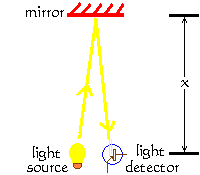A light-clock comprises a light source, a mirror mounted a fixed distance x away from the source and a detector next to the light source. This arrangement is illustrated on the right. The light source is triggered electronically to emit a very short pulse of light. The light travels to the mirror from where it is reflected back to the detector. The dete­ctor emits an electrical pulse that triggers the light source to emit the next pulse of light. The period of the clock, that is, the duration of the clock-tick, is the amount of time it takes the light to travel the distance 2x to the mirror and back. We must make the distance x very large so that the delay in the electronic trigger is negligible in comparison with the time taken by the light to travel to the mirror and back. The diagram shows the outbound and return paths of the light pulse at a slight angle to each other. This is just to make the illustration clear. It is in­tended that the light source and the detector should be as coincident as possible, thus making the return path follow exactly the same line as the outbound path.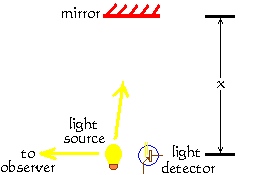The same light source that sends the pulse of light in the direction of the mirror also sends a pulse of light towards the observer, as shown on the left. The emission of this pulse marks the start of a new tick of the clock. Later, when the light pulse returns from the mirror and enters the de­tector, the dete­ctor triggers the light source again to send another pulse. The emission of this sec­ond pulse marks the end of this clock tick and the start of the next one. This second pulse also trav­els towards the observ­er, arriving somewhat later.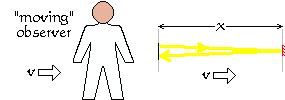Let us now turn the clock horizontal as shown on the right. The diagram of the clock has been simplified. An observer watches the clock's elec­tronic counter as it counts the clock ticks. The observer is stationary with re­s­pect to the clock. Whether the observer and the clock are stat­ionary or moving together at the same velocity v is irrelevant to the period of the clock tick ob­served by the observer.

Ignoring the "negligible" delay in the clock's electronics, the period of the clock tick will be 2x/c seconds. Now suppose the clock is moving away from the observer at velocity v, as depicted below.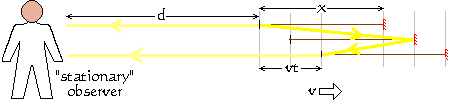At the instant the light pulse leaves the light source, information about this event leaves the vicinity of the light source also. This information traverses the distance d to the observer at c, the velocity of light.

In the observer's frame of reference, we can see that by the time the light reaches the mirror, the mirror has travelled a distance ½(vt) further on. The mirror reflects the light. But now it hasn't far to go. This is because, by the end of the clock period t, the light detector has travelled a distance vt further away from the observer. Nevertheless, the actual distance travelled by the light (in the observer's frame of reference) is still 2x, as it was for the "moving" observer in the case above. Check it out. To get to the mirror, the light travels a distance x + ½(vt). To get back from the mirror to the detector, it travels a further distance of x - ½(vt). So the total distance it travels is 2x. So there is no need for contracted space or dilated time here.

When the light pulse arrives at the light detector, information of this event embarks on its journey from the detector to the observer. This information, however, must travel a distance of d + vt. So the information advising the observer of the end of the clock-tick must travel a distance vt further than the information that advised him of the start of the clock-tick. This means that the period of the clock-tick from the point of view of the observer is (2x + vt)/c seconds instead of the simple 2x/c seconds as it was for the "moving" observer.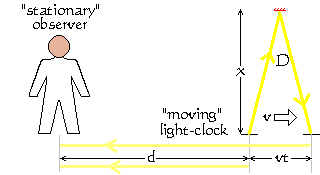Let us now turn the "moving" light-clock so that its light pulse travels to the mirror and back in a direction perpendicular to the dir­ection of the "stationary" observer. Consid­er the path of the clock's light pul­se within the observer's frame of refer­en­ce. By the time the light pulse from the source arrives at the mirror, the mirror itself has moved a distance ½(vt) further to the right. Then, by the time the ref­lected light pulse has made the return trip and has reached the detector, the detector itself has moved the full distance of vt from where it was when the light pulse originally left the source.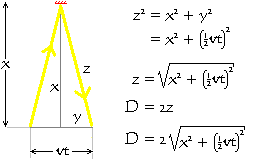This means that, within the "stationary" obser­ver's frame of reference, the clock's light pulse travels the distance D given by the formula shown in the diagram on the left. This formula is derived by applying Pythagoras's Theorem to one half of the isosceles triangle formed by the path of the light pulse and the base line, and then doubling the result.

From this reasoning, it would seem that the tick-period of the light-clock, as per­ceived by the "stationary" observer, would be (D + vt)/c seconds.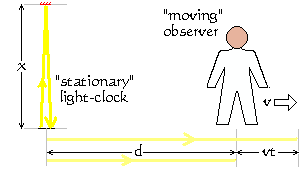Velocity is a relative concept. This means we are free to swap whom we consider to be "stationary" and whom we consider to be "moving". So now consider the light-clock to be "stationary" and the observer to be "moving" relative to the light-clock, as shown on the right. The difference in the arrival times at the observer between the signals marking the start and end of a clock-tick is now only (2x + vt)/c seconds.

The quantitative difference between this period and the period (D + vt)/c (for the "moving" clock obtained above) is not important. What is important is simply that they are different.

One could argue that the reason for the difference is that we are considering the situation from the points of view of two different frames of reference. However, if there were a million different observers each travelling at a different velocity in a different direction with respect to the light-clock, there would be a million different values of D for the passage of the light within the one single clock. Each observer would also perceive a different period for the clock-tick when the "moving" clock is in line with him from when it is perpendicular to him, or at some other angle.

Does the presence of all these observers thus contract the space and dilate the time within the vicinity of this single clock? Does this light-clock effectively exist in a different superposition of state within a million different universes?

With respect to the light-clock, an observer is passive. For all practical purposes, he has no effect on the clock. Consequently, the transit of the light from the source to the mirror and back to the detector is in no way influenced by his presence or the presence of however many observers we may wish to consider. Furthermore, dur­ing its journey from the source to the mirror and back to the detector, the light within the light-clock is unobservable by the observer. The light is born, lives and dies within the confines of the clock. Any observer can only observe information signals (experience the arrival of light waves) that are travelling directly towards him. Such phenomena travelling in any other direction are not part of his universe of experi­ence.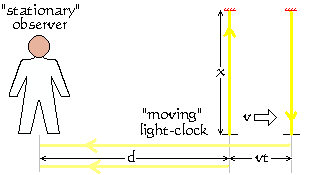The fact that the observer is continually changing his position — and therefore his point of view — while the light is travel­ling between the source, mirror and det­ect­or does not in any way influence the motion of the light. Just because the obs­erver perceives the clock to be moving side­ways does not mean the light itself has a real sideways component to its mo­tion. This means that, from whoever's point of view we consider the motion of the clock and the observer, the light still really travels only a distance 2x within the clock, in a time t = 2x/c.

The communication of information to the observer about the start time and stop time of one clock-tick is an entirely separate physical transaction. The light used for the light-clock and the light used to signal to the observer have the same source. However the light received by the observer travels in a different direction from the light used by the light-clock. The light within the light-clock is unobservable by the observer. He has no notion of its existence or its journey. He is fundamentally un­able to have any notion of its existence or its journey. It is outside his universe of experience. It is outside his frame of reference. Only light travelling along paths that intersect his position in space and time can be detected and experienced by him. He can have no knowledge or connection with light travelling elsewhere.

The Observer is Passive

An observer has no way of sensing the approach of a light-pulse. He has no way of sensing when it left its source. He has no way of sensing how far it has travelled. He has no way of sensing how fast it is travelling towards him. He can only sense it when it eventually "hits" him. Even then, he has no way to sense its velocity of im­pact.

The only thing an observer can experience as the result of an arriving light-pulse is a forced change in the electric or magnetic field-strength in his immediate vicinity. And this change of field strength is actually perpendicular (at right-angles) to the direction from which the light came. Consequently, since the transverse wave mo­tion of light does not involve any material movement in the direction the wave is "travelling", the notion of its having an "impact" velocity with the observer is essen­tially meaningless.

An observer can therefore deduce nothing within his frame of reference about the velocity the light had during its journey. He had no control or influence over the source of the light at the time the light was created. Consequently, the light an observer receives has no reason or motivation to tie its velocity in any way to an observer's frame of reference.

This reinforces the notion that the only frame of reference within which light travels at velocity c is that of its source.

A Source-Centred View

Let us return to consider the observer observing a source of light. Again, it is mov­ing away from him at a significant fraction of the velocity of light. As before, in free space we know that velocity is relative. Consequently, it should not matter whether we think of the observer as "stationary" and the light-source as "moving" or whe­ther we think of the light-source as "stationary" and the observer as "moving". Whichever of these two points of view we choose should, in principle, make no diff­erence to the way light travels between the light-source and the observer.

Thus we can no longer accept that light travels with velocity c in all frames of ref­erence. Instead, we must accept that light travels with velocity c only relative to its source. If we accept this, then for any observer of the light-source, these two points of view will be as follows.

1) Moving Light-Source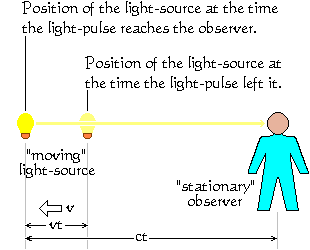The case where we consider the source of light to be moving is shown on the right. A pulse of light travels from the "origin" of the source's frame of reference where it was emitted. It traverses the space from that point to where the observer is located when the light reaches him. It traverses this space at velocity c in time t. The dist­ance it travels is therefore ct. During the time t, the "mov­ing" light-source travels a distance vt further from the observer, where v is the relative velocity between the observer and the light-source. At the time the light-pulse reaches the observer, the observer will see it as hav­ing come from the position where the light-source actually is at that time. However, the observer will then see the light-source as it was when the light-pulse left it. He will see it as it appeared t (units of time) in the past.

2) Moving ObserverOn the left is shown the reverse situation where we consider the source of light to be "stationary" and the observer to be "mov­ing". A light-pulse is emitted by the light-source. It travels at velocity c towards the observer. While the light-pulse is travelling towards the observer, the observer is mov­ing ever-further away from the light-source at velocity v. Thus, by the time the pulse of light reaches the observer, he has travelled a distance vt further away from the light-source. During this same amount of time t, the light-pulse has travelled a distance ct. So in this situation, the distan­ce between the reality of the light-source and the observer is again ct. The obser­ver again per­ceives the light-source to be "now" where it actually is "now", although he sees it as it was at an amount of time t in the past.

So in this way of viewing the process, the two above points of view are identical. There is no paradox. This source-centred view also circumvents the paradox of the "perpendicular" light-clock, where the period of the clock would be different accord­ing to which is regarded as "stationary" — the observer or the clock.

This would lead us to consider light simply as part of its source. A light-source is a microscopic structure of captured energy. When it emits light, the source disperses (casts off) part of its essence into the rest of universe as a diverging electromag­netic disturbance. The velocity with which this disturbance travels must therefore be relative to its source.

This suggests that light must be, essentially, a dispersing extension of its source. Before the light is emitted, its energy is part of that held captive within the struc­ture of its source. After the light is emitted, its energy is released into the universe as an ever-expanding travelling disturbance. The amount of energy in this disturb­ance is the amount of energy released by the source. From this, it would seem reasonable to suppose that the quantity of energy released by the source, in any given trans­action of emission, would be determined solely by the nature, structure and mech­anism of its microscopic source.

Although the source-centred view appears to work for light, it is not consistent with any view of other observable phenomena such as gravity. Notwithstanding, there is another way of constructing an observer-centred view of light, which is consistent with a complementary view of gravity.

The Æthereal View

In my nine essays about The Universe, I gradually built up an observer-centred view based on the notion that the universe is made of an all-pervading velocity fluid, which I refer to as the æther. I call it a velocity fluid because it can only exist while travelling at the speed of light. Furthermore, it only manifests itself to objects that are accelerating. It does not interact in any way with objects travelling at con­stant relative velocity. It flows convergently, at the speed of light, into nanoscopic sink-holes at the centres of all fundamental constituents of matter.

In this view, which is expounded fully in my essay entitled Events and Waves, a light-source simply etches electromagnetic stresses into the observer's passing æthereal influx. The passing æther flows radially inwards towards the observer at the speed of light, carrying the electromagnetic etchings with it. Necessarily, in this view, the velocity of light, c, is well and truly tied to the observer.

Notwithstanding, if we simply transpose the above source-centric view [described above] to an observer-centric one, the geometry and mathematics do not change and the light-clock paradox disappears. All it requires is that, when the source etches its inscription onto the passing æther, that etching is no longer bound to travel from the source at velocity c. Instead, it must always travel towards the ob­server at velocity c.

© 13 September 2006 Robert John Morton | PREV | NEXT